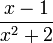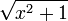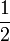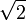# Polynomial

In algebra, a polynomial is, roughly speaking, a formal expression obtained from constants and one or several variables by making a finite number of additions, subtractions and multiplications. For instance, x2 − 2x + 1 is a polynomial involving one variable, x (often called a polynomial in one variable), whereas x2 + y2 is a polynomial in two variables, x and y.

Polynomials are an essential element of most applications of mathematics to a systematic study of physical problems and phenomena. Polynomials are the most basic objects that can be used to represent situations where a certain quantity is unknown, like the time it will take for a ball thrown in the air to fall back to the ground. They are also the most basic objects that can be used to represent a quantity that varies. For instance, the rate at which a chemical reaction proceeds often varies as the concentrations of the reactants change. This variation is often specified by a polynomial whose variables are the concentrations.

The study of many phenomena requires more sophisticated objects than polynomials. Sometimes such analysis is so complicated that it becomes essential to approximate certain objects with polynomials. Approximation of sophisticated mathematical objects with polynomials is an important application of calculus, and is an essential tool in numerical analysis.

The polynomials encountered first in school and occurring most commonly in applications have real number coefficients. However, there are applications where other types of coefficients occur. For instance, the study of very small phenomena using quantum mechanics requires the use of complex numbers. Error-correcting codes, used to avoid loss or corruption of data, involve coefficients governed by arithmetic modulo 2. Polynomials with complex number coefficients or coefficients modulo 2 behave similarly to polynomials with real number coefficients in many ways. The similarities and differences are discussed on the advanced version of this page. Polynomials with even more general types of coefficients are important in advanced mathematics, and are discussed on the polynomial ring page. For the rest of the present article, all polynomials considered will have real number coefficients.

##  Non-Examples

Expressions like$\frac{x-1}{x^2+2}$ or$\sqrt{x^2+1}$ are not polynomials ; the first one is a rational function, because of the fraction bar (representing division), and the second one is an irrational expression, because of the square root symbol. Such operations might be expressed within the constant numbers, as in the example$\frac{1}{2}x^3+x-\sqrt{2}$, but this is only because$\frac{1}{2}$ and$\sqrt{2}$ are elements of the set (e.g. real numbers) that are being used as coefficients of the polynomials.

Expressions involving exponential functions, like 2x + 1, are often mistaken for polynomials because of the similarity of notation. It is important to realize that the exponents appearing in a polynomial are always constants; when an expression has a variable in an exponent, such as the exponent x on the base 2 in the above expression, the expression is not a polynomial.

##  Monomials and simplification

The simplest polynomials are the monomials having only one term. A monomial is either a constant, or a product of constants and positive whole number powers of variables. The constant is called the coefficient of the monomial. A coefficient equal to 1 is typically dropped from the notation, so that x2 represents the same monomial as 1x2. Coefficients are usually written in as simplified a form as possible, but even then can sometimes look rather complicated, such as the coefficient in$(\pi + \sqrt{3} + 1)x$. When a coefficient involves a sum, parentheses must be inserted around the coefficient.

Monomials are usually immediately simplified so that each variable is written once. For instance, x2yx would be simplified by combing both powers of the variable x, producing x3y. When a monomial involves several variables, the order in which the variables are written is unimportant. By convention, the variables are typically listed in alphabetical order. For instance, x3y and yx3 are considered to be the same monomial, but the latter would usually be rewritten as the former.

Polynomials are constructed by adding together a finite number of monomials. For instance, 1, 2x4, and − 3x2yz3 are monomials, and their sum, 1 + 2x4 − 3x2yz3 is a polynomial which is not a monomial.

There are two main procedures used in simplifying a polynomial. First, one can remove groupings by distributing, which produces a sum of monomials. For instance, 2x(x + 2) + x(2x + 2) simplifies to 2x2 + 4x + 2x2 + 2x. Second, one can combine like terms to reduce the number of monomials in the expression. For instance, the above polynomial can be further simplified to 4x2 + 6x by combining the two terms involving x2 and the two terms involving x.

##  Polynomials in one variable

In this section we deal with the simplest case, that is, polynomials involving only one variable, denoted x.

### Degree and the Standard Form

After simplification, a polynomial can be written as a finite sum of monomials. It is sometimes useful to explicitly write a power of a variable in each monomial, even the constants. To accomplish this, you can write x0 after the constant, so that 2 and 2x0 are considered the same.

The power of the variable appearing in a monomial is the degree of the monomial. By the above convention, a constant c is the same as cx0 and has degree equal to 0. The degree of a polynomial is the largest of the degrees of the monomials appearing in the polynomial. The only exception is the constant polynomial 0, which typically is not assigned a degree (for reasons made clear below). As an example, 2 has degree 0, x2 + x has degree 2, and − 3x4 + x2 + x5 has degree 5.

The degree is an important identifier when working with polynomials. For instance, many procedures for factoring or solving polynomial equations require identifying the degree of the polynomial first. In the last example above, we had to scan through the polynomial from the left all the way through the right to determine that the degree is 5. To facilitate identifying the degree of a polynomial, as well as manipulations of polynomials, they are usually written in standard form. The standard form of a polynomial is obtained by simplifying as described above and then writing the monomials so that the exponents decrease from left to right. The degree of a polynomial in standard form is the degree of the first monomial appearing. The term of highest degree is the leading term and its coefficient is the leading coefficient. A polynomial with leading coefficient equal to 1 is monic. We can put the last example above in standard form by rearranging the monomials to obtain x5 − 3x4 + x2. It is of course just as easy to work with polynomials where the monomials are written so that the degrees increase from left to right.

##  Applications of polynomialsSome content on this page may previously have appeared on Citizendium.